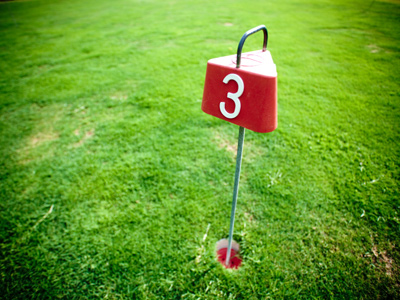A quarter of 12 is 3.

# Fractions 1

This Math quiz is called 'Fractions 1' and it has been written by teachers to help you if you are studying the subject at elementary school. Playing educational quizzes is an enjoyable way to learn if you are in the 3rd, 4th or 5th grade - aged 8 to 11.

It costs only \$12.50 per month to play this quiz and over 3,500 others that help you with your school work. You can subscribe on the page at Join Us

If you cut a cake up to share amongst your friends you are dividing it into fractions. Fractions are numbers which are less than a whole. You may know that one third is written as 13 in fraction form, or that three quarters is 34. But how good are you at recognizing their values? How many thirds or quarters make a whole? And which is bigger - two thirds or a half?

Practice what you have learned in your Math classes so far by playing this quiz all about fractions and see how good you are at recognizing their values.

1.
One third and which other fraction make a whole one?
210
12
34
23
13 + 23 = 33 which is the same as 1
2.
Which represents one third?
14
12
13
15
13 is the same as 1 ÷ 3
3.
What does 110 mean?
One whole one divided into ten equal parts
Ten whole ones divided equally
Five whole ones divided equally
One whole one divided into two equal parts
110 is the same as 1 ÷ 10
4.
Which of these is equivalent to 510?
12
23
34
14
If you divide 5 by 5 you get 1 and if you divide 10 by 5 you get to so 510 is the same as 12
5.
Which is the largest of these fractions?
12
510
23
24
12 is the same as 510 and 24
6.
How many tenths in one whole?
5
10
2
4
A tenth is one part when the whole is divided into ten equal parts
7.
Which fraction lies exactly between 12 and 1?
14
710
23
34
12 = 24 and 1 = 44 so 34 is between the two
8.
What is a quarter of 12?
3
4
6
9
To calculate a quarter divide by 4
9.
If we have 34 what is needed to make a whole?
12
13
14
110
34 plus 14 = 44 which is one whole
10.
Which is the smallest of these fractions?
12
14
13
110
If the numbers above the line are the same then the higher the number below the line the lower the fraction is
Author:  Amanda Swift